Olympiad Test: Embedded Figures - 2

# Olympiad Test: Embedded Figures - 2

Test Description

## 10 Questions MCQ Test National Cyber Olympiad Class 6 | Olympiad Test: Embedded Figures - 2

Olympiad Test: Embedded Figures - 2 for Class 6 2022 is part of National Cyber Olympiad Class 6 preparation. The Olympiad Test: Embedded Figures - 2 questions and answers have been prepared according to the Class 6 exam syllabus.The Olympiad Test: Embedded Figures - 2 MCQs are made for Class 6 2022 Exam. Find important definitions, questions, notes, meanings, examples, exercises, MCQs and online tests for Olympiad Test: Embedded Figures - 2 below.
Solutions of Olympiad Test: Embedded Figures - 2 questions in English are available as part of our National Cyber Olympiad Class 6 for Class 6 & Olympiad Test: Embedded Figures - 2 solutions in Hindi for National Cyber Olympiad Class 6 course. Download more important topics, notes, lectures and mock test series for Class 6 Exam by signing up for free. Attempt Olympiad Test: Embedded Figures - 2 | 10 questions in 20 minutes | Mock test for Class 6 preparation | Free important questions MCQ to study National Cyber Olympiad Class 6 for Class 6 Exam | Download free PDF with solutions
 1 Crore+ students have signed up on EduRev. Have you?
Olympiad Test: Embedded Figures - 2 - Question 1

### Directions: In each of the following questions, you are given a figure (X) followed by four alternative figures (A), (B), (C) and (D) such that figure (X) is embedded in one of them. Find out the alternative figure which contains fig. (X) as its part. Q. Find out the alternative figure which contains figure (X) as its part.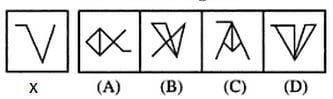Olympiad Test: Embedded Figures - 2 - Question 2

### Directions: In each of the following questions, you are given a figure (X) followed by four alternative figures (A), (B), (C) and (D) such that figure (X) is embedded in one of them. Find out the alternative figure which contains fig. (X) as its part. Q. Find out the alternative figure which contains figure (X) as its part.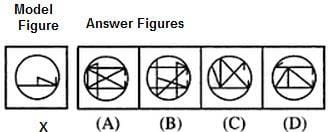Olympiad Test: Embedded Figures - 2 - Question 3

### Directions: In each of the following questions, you are given a figure (X) followed by four alternative figures (A), (B), (C) and (D) such that figure (X) is embedded in one of them. Find out the alternative figure which contains fig. (X) as its part. Q. Find out the alternative figure which contains figure (X) as its part.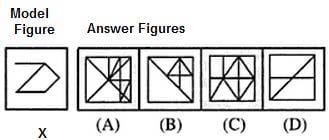Olympiad Test: Embedded Figures - 2 - Question 4

Directions: In each of the following questions, you are given a figure (X) followed by four alternative figures (A), (B), (C) and (D) such that figure (X) is embedded in one of them. Find out the alternative figure which contains fig. (X) as its part.

Q. Find out the alternative figure which contains figure (X) as its part.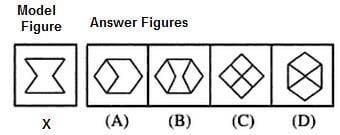Olympiad Test: Embedded Figures - 2 - Question 5

Directions: In each of the following questions, you are given a figure (X) followed by four alternative figures (A), (B), (C) and (D) such that figure (X) is embedded in one of them. Find out the alternative figure which contains fig. (X) as its part.

Q. Find out the alternative figure which contains figure (X) as its part.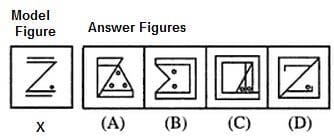Olympiad Test: Embedded Figures - 2 - Question 6

Directions: In each of the following questions, you are given a figure (X) followed by four alternative figures (A), (B), (C) and (D) such that figure (X) is embedded in one of them. Find out the alternative figure which contains fig. (X) as its part.

Q. Find out the alternative figure which contains figure (X) as its part.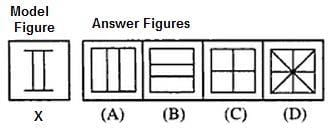Olympiad Test: Embedded Figures - 2 - Question 7

Directions: In each of the following questions, you are given a figure (X) followed by four alternative figures (A), (B), (C) and (D) such that figure (X) is embedded in one of them. Find out the alternative figure which contains fig. (X) as its part.

Q. Find out the alternative figure which contains figure (X) as its part.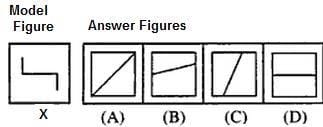Olympiad Test: Embedded Figures - 2 - Question 8

Directions: In each of the following questions, you are given a figure (X) followed by four alternative figures (A), (B), (C) and (D) such that figure (X) is embedded in one of them. Find out the alternative figure which contains fig. (X) as its part.

Q. Find out the alternative figure which contains figure (X) as its part.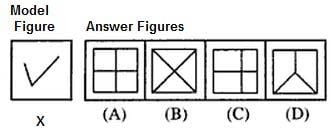Olympiad Test: Embedded Figures - 2 - Question 9

Directions: In each of the following questions, you are given a figure (X) followed by four alternative figures (A), (B), (C) and (D) such that figure (X) is embedded in one of them. Find out the alternative figure which contains fig. (X) as its part.

Q. Find out the alternative figure which contains figure (X) as its part.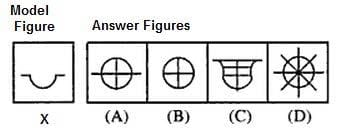Olympiad Test: Embedded Figures - 2 - Question 10

Directions: In each of the following questions, you are given a figure (X) followed by four alternative figures (A), (B), (C) and (D) such that figure (X) is embedded in one of them. Find out the alternative figure which contains fig. (X) as its part.

Q. Find out the alternative figure which contains figure (X) as its part.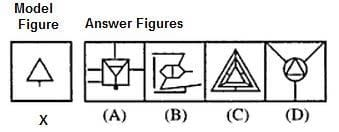## National Cyber Olympiad Class 6

34 tests
 Use Code STAYHOME200 and get INR 200 additional OFF Use Coupon Code
Information about Olympiad Test: Embedded Figures - 2 Page
In this test you can find the Exam questions for Olympiad Test: Embedded Figures - 2 solved & explained in the simplest way possible. Besides giving Questions and answers for Olympiad Test: Embedded Figures - 2, EduRev gives you an ample number of Online tests for practice

34 tests